Anzeige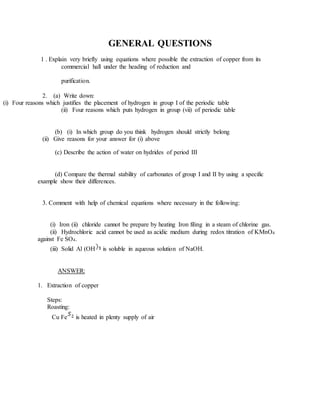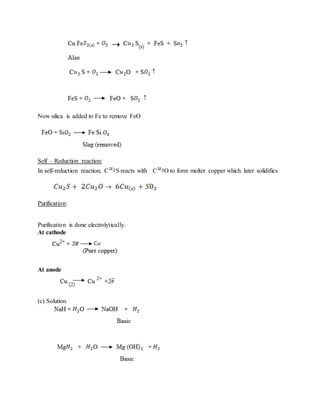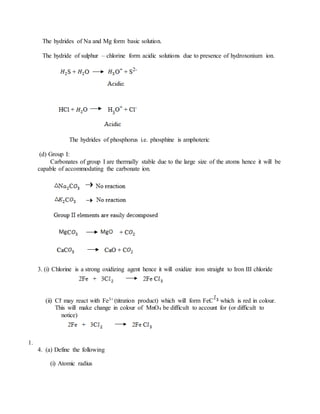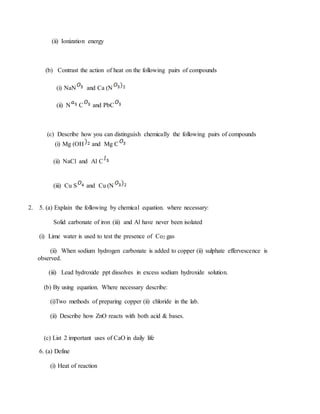Anzeige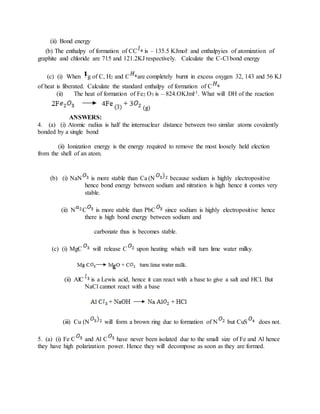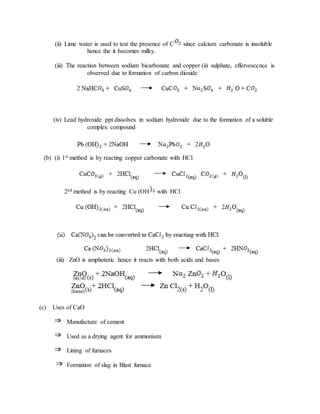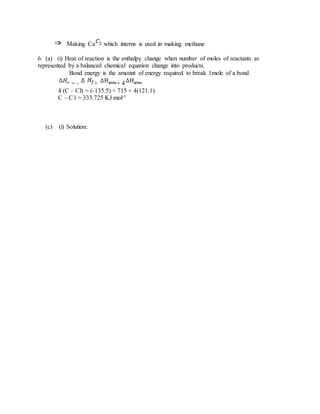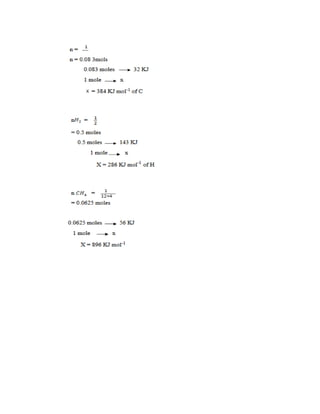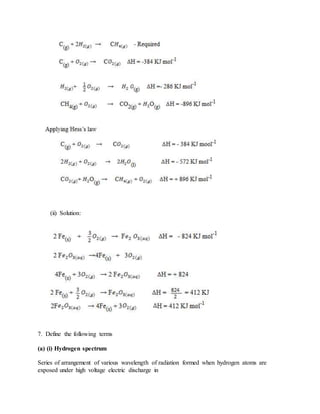Anzeige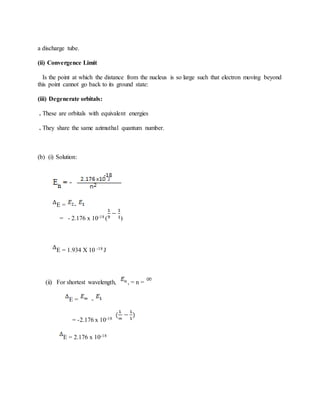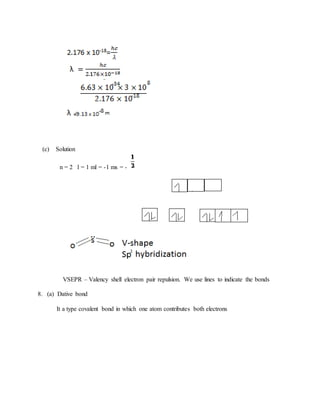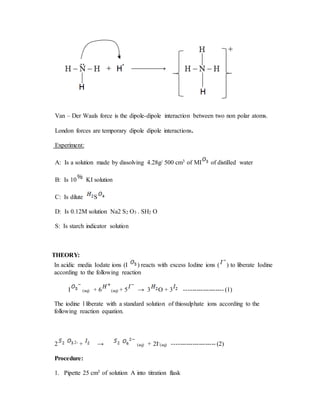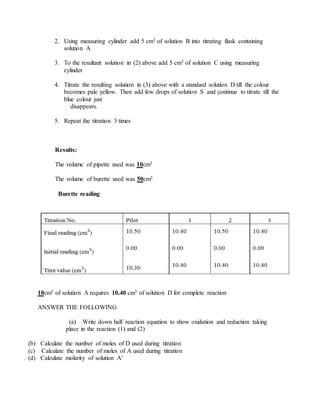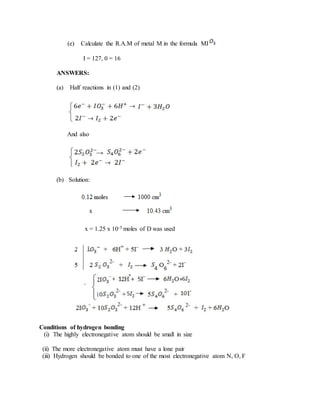Anzeige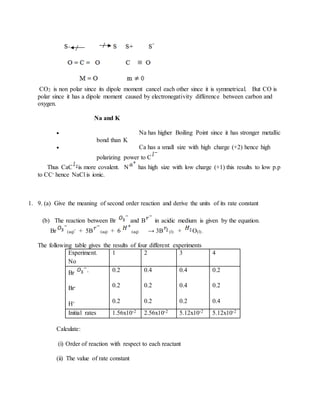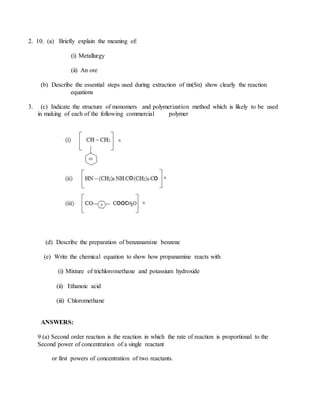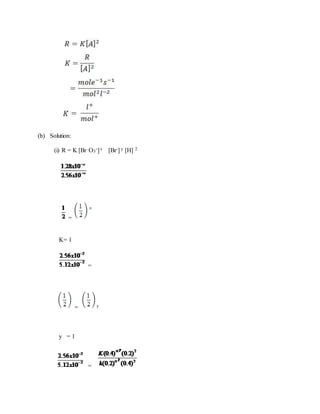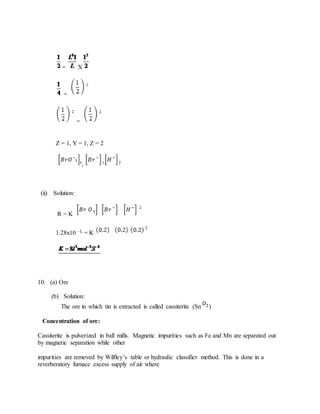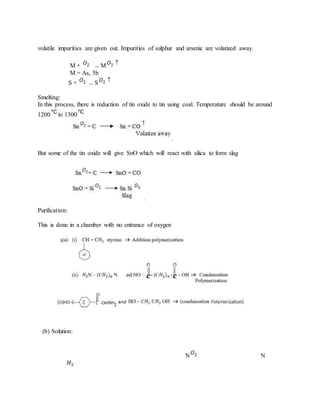Anzeige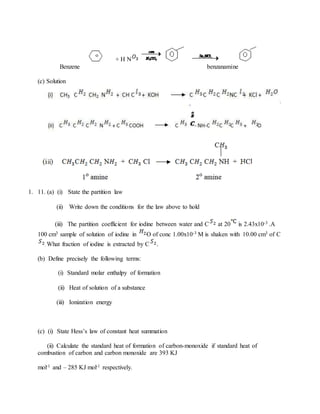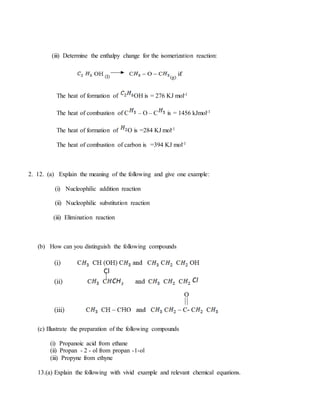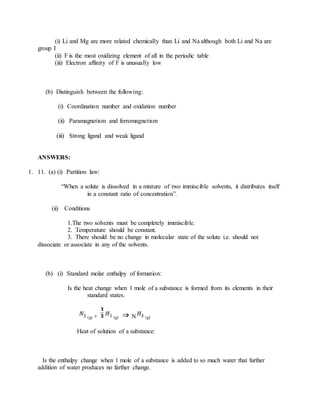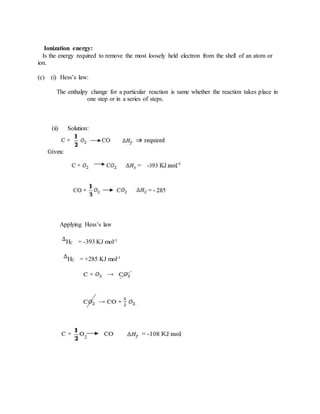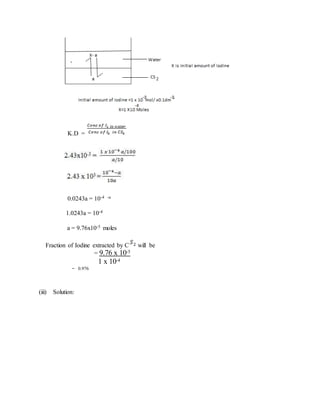Anzeige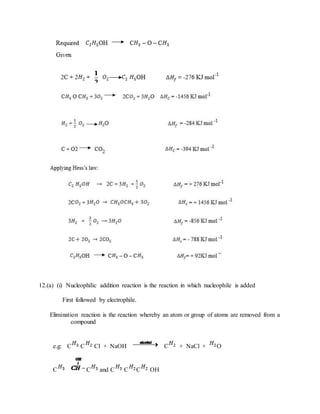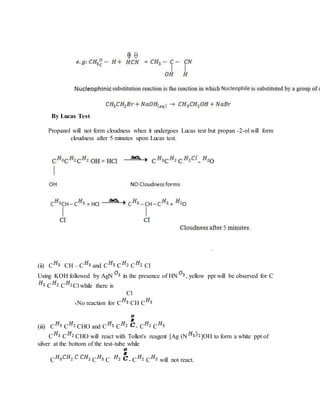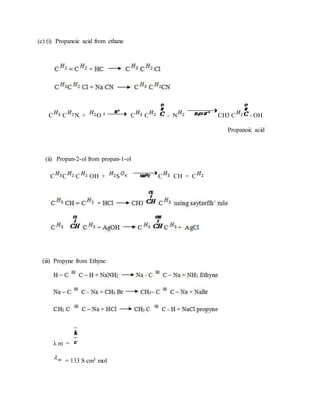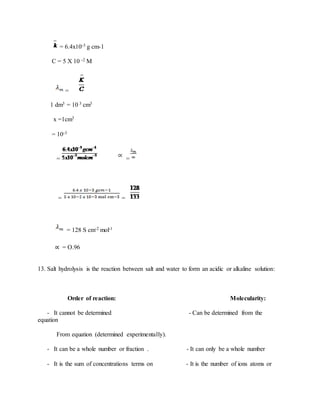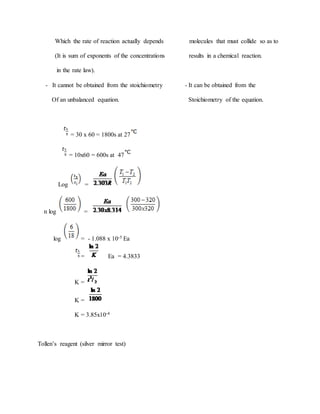Anzeige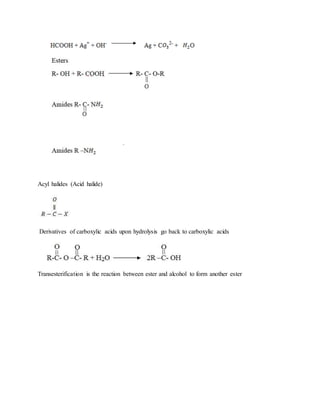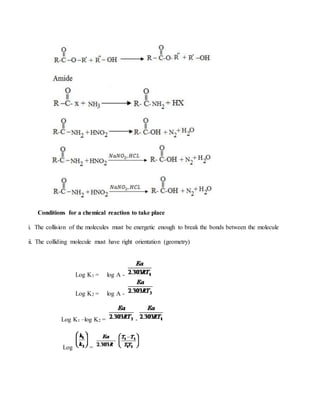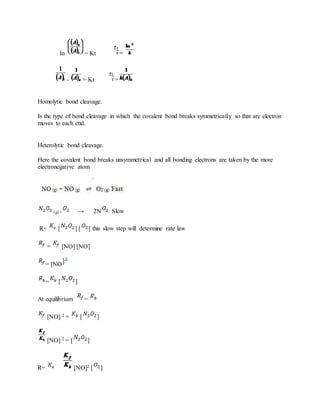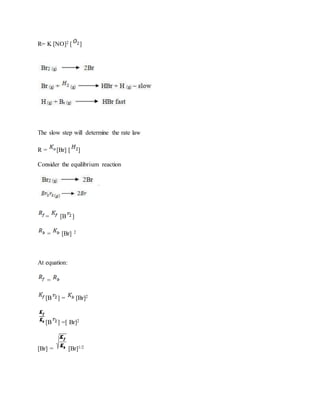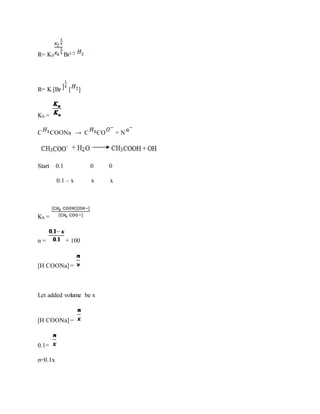Anzeige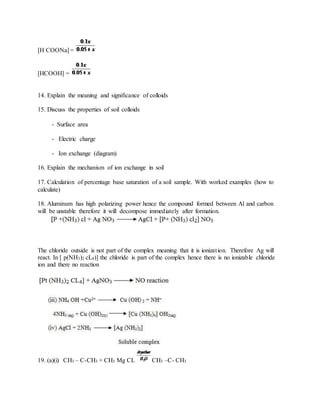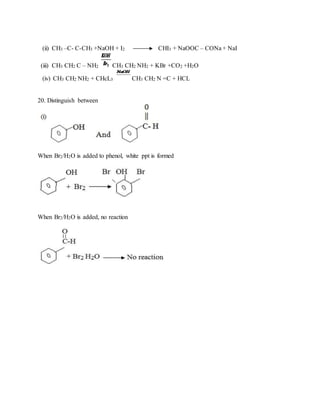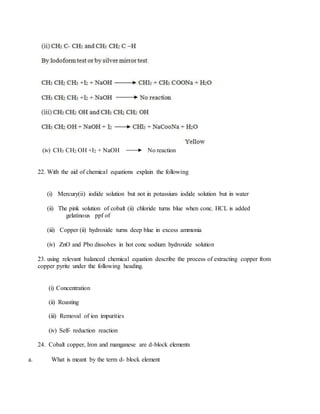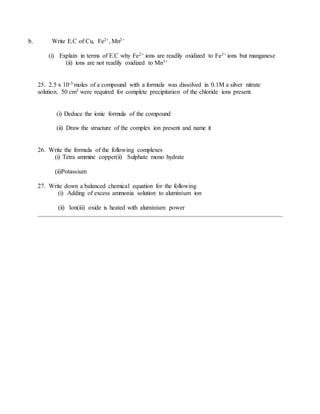Nächste SlideShare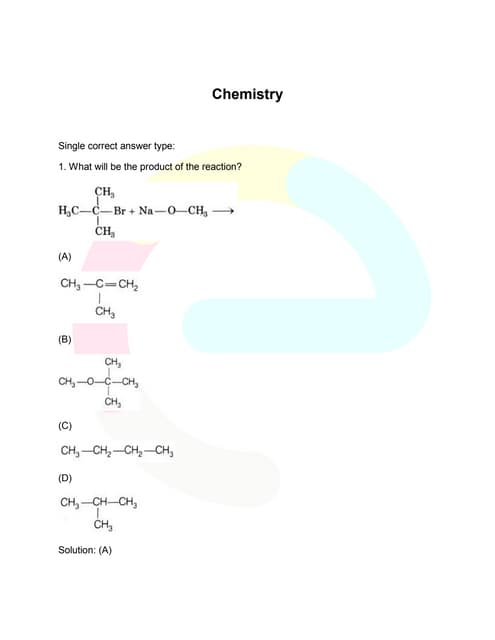Bitsat previous-year-question-papers-2014
1 von 38
Anzeige

### GENERAL QUESTIONS

1. GENERAL QUESTIONS 1 . Explain very briefly using equations where possible the extraction of copper from its commercial hall under the heading of reduction and purification. 2. (a) Write down: (i) Four reasons which justifies the placement of hydrogen in group I of the periodic table (ii) Four reasons which puts hydrogen in group (vii) of periodic table (b) (i) In which group do you think hydrogen should strictly belong (ii) Give reasons for your answer for (i) above (c) Describe the action of water on hydrides of period III (d) Compare the thermal stability of carbonates of group I and II by using a specific example show their differences. 3. Comment with help of chemical equations where necessary in the following: (i) Iron (ii) chloride cannot be prepare by heating Iron filing in a steam of chlorine gas. (ii) Hydrochloric acid cannot be used as acidic medium during redox titration of KMnO4 against Fe SO4. (iii) Solid Al (OH is soluble in aqueous solution of NaOH. ANSWER: 1. Extraction of copper Steps: Roasting: Cu Fe is heated in plenty supply of air
2. Now silica is added to Fe to remove FeO Self – Reduction reaction: In self-reduction reaction, C S reacts with C O to form molter copper which later solidifies Purification: Purification is done electrolytically. At cathode At anode (c) Solution.
3. The hydrides of Na and Mg form basic solution. The hydride of sulphur – chlorine form acidic solutions due to presence of hydroxonium ion. The hydrides of phosphorus i.e. phosphine is amphoteric (d) Group I: Carbonates of group I are thermally stable due to the large size of the atoms hence it will be capable of accommodating the carbonate ion. 3. (i) Chlorine is a strong oxidizing agent hence it will oxidize iron straight to Iron III chloride (ii) Cl- may react with Fe3+ (titration product) which will form FeC which is red in colour. This will make change in colour of MnO4 be difficult to account for (or difficult to notice) 1. 4. (a) Define the following (i) Atomic radius
4. (ii) Ionization energy (b) Contrast the action of heat on the following pairs of compounds (i) NaN and Ca (N (ii) N C and PbC (c) Describe how you can distinguish chemically the following pairs of compounds (i) Mg (OH and Mg C (ii) NaCl and Al C (iii) Cu S and Cu (N 2. 5. (a) Explain the following by chemical equation. where necessary: Solid carbonate of iron (iii) and Al have never been isolated (i) Lime water is used to test the presence of Co2 gas (ii) When sodium hydrogen carbonate is added to copper (ii) sulphate effervescence is observed. (iii) Lead hydroxide ppt dissolves in excess sodium hydroxide solution. (b) By using equation. Where necessary describe: (i)Two methods of preparing copper (ii) chloride in the lab. (ii) Describe how ZnO reacts with both acid & bases. (c) List 2 important uses of CaO in daily life 6. (a) Define (i) Heat of reaction
5. (ii) Bond energy (b) The enthalpy of formation of CC is – 135.5 KJmol- and enthalpyies of atomization of graphite and chloride are 715 and 121.2KJ respectively. Calculate the C-Cl bond energy (c) (i) When g of C, H2 and C are completely burnt in excess oxygen 32, 143 and 56 KJ of heat is liberated. Calculate the standard enthalpy of formation of C (ii) The heat of formation of Fe2 O3 is – 824.OKJml-1. What will DH of the reaction ANSWERS: 4. (a) (i) Atomic radius is half the internuclear distance between two similar atoms covalently bonded by a single bond (ii) Ionization energy is the energy required to remove the most loosely held election from the shell of an atom. (b) (i) NaN is more stable than Ca (N because sodium is highly electropositive hence bond energy between sodium and nitration is high hence it comes very stable. (ii) N C is more stable than PbC since sodium is highly electropositive hence there is high bond energy between sodium and carbonate thus is becomes stable. (c) (i) MgC will release C upon heating which will turn lime water milky. (ii) AlC is a Lewis acid, hence it can react with a base to give a salt and HCl. But NaCl cannot react with a base (iii) Cu (N will form a brown ring due to formation of N but CuS does not. 5. (a) (i) Fe C and Al C have never been isolated due to the small size of Fe and Al hence they have high polarization power. Hence they will decompose as soon as they are formed.
6. (ii) Lime water is used to test the presence of C since calcium carbonate is insoluble hence the it becomes milky. (iii) The reaction between sodium bicarbonate and copper (ii) sulphate, effervescence is observed due to formation of carbon dioxide (iv) Lead hydroxide ppt dissolves in sodium hydroxide due to the formation of a soluble complex compound (b) (i) 1st method is by reacting copper carbonate with HCl 2nd method is by reacting Cu (OH with HCl (iii) ZnO is amphoteric hence it reacts with both acids and bases (c) Uses of CaO Manufacture of cement Used as a drying agent for ammonium Lining of furnaces Formation of slag in Blast furnace
7. Making Ca which interns is used in making methane 6. (a) (i) Heat of reaction is the enthalpy change when number of moles of reactants as represented by a balanced chemical equation change into products. Bond energy is the amount of energy required to break 1mole of a bond = - + + 4 4 (C – Cl) = (-135.5) + 715 + 4(121.1) C – C l = 333.725 KJ mol-1 (c) (i) Solution:
8. (ii) Solution: 7. Define the following terms (a) (i) Hydrogen spectrum Series of arrangement of various wavelength of radiation formed when hydrogen atoms are exposed under high voltage electric discharge in
9. a discharge tube. (ii) Convergence Limit Is the point at which the distance from the nucleus is so large such that electron moving beyond this point cannot go back to its ground state: (iii) Degenerate orbitals: . These are orbitals with equivalent energies . They share the same azimuthal quantum number. (b) (i) Solution: E = - = - 2.176 x 10-18 ( ) E = 1.934 X 10 -18 J (ii) For shortest wavelength, , = n = E = - = -2.176 x 10-18 E = 2.176 x 10-18
10. (c) Solution n = 2 l = 1 ml = -1 ms = - VSEPR – Valency shell electron pair repulsion. We use lines to indicate the bonds 8. (a) Dative bond It a type covalent bond in which one atom contributes both electrons
11. Van – Der Waals force is the dipole-dipole interaction between two non polar atoms. London forces are temporary dipole dipole interactions. Experiment: A: Is a solution made by dissolving 4.28g/ 500 cm3 of MI of distilled water B: Is 10 KI solution C: Is dilute S D: Is 0.12M solution Na2 S2 O3 . SH2 O S: Is starch indicator solution THEORY: In acidic media Iodate ions (I ) reacts with excess Iodine ions ( ) to liberate Iodine according to the following reaction I (aq) + 6 (aq) + 5 → 3 O + 3 ------------------- (1) The iodine l liberate with a standard solution of thiosulphate ions according to the following reaction equation. 2 2- + → (aq) + 2I- (aq) --------------------- (2) Procedure: 1. Pipette 25 cm3 of solution A into titration flask
12. 2. Using measuring cylinder add 5 cm3 of solution B into titrating flask containing solution A 3. To the resultant solution in (2) above add 5 cm5 of solution C using measuring cylinder 4. Titrate the resulting solution in (3) above with a standard solution D till the colour becomes pale yellow. Then add few drops of solution S and continue to titrate till the blue colour just disappears. 5. Repeat the titration 3 times Results: The volume of pipette used was 10cm3 The volume of burette used was 50cm3 Burette reading 10cm3 of solution A requires 10.40 cm3 of solution D for complete reaction ANSWER THE FOLLOWING (a) Write down half reaction equation to show oxidation and reduction taking place in the reaction (1) and (2) (b) Calculate the number of moles of D used during titration (c) Calculate the number of moles of A used during titration (d) Calculate molarity of solution A’
13. (e) Calculate the R.A.M of metal M in the formula MI I = 127, 0 = 16 ANSWERS: (a) Half reactions in (1) and (2) And also (b) Solution: x = 1.25 x 10-3 moles of D was used Conditions of hydrogen bonding (i) The highly electronegative atom should be small in size (ii) The more electronegative atom must have a lone pair (iii) Hydrogen should be bonded to one of the most electronegative atom N, O, F
14. CO2 is non polar since its dipole moment cancel each other since it is symmetrical. But CO is polar since it has a dipole moment caused by electronegativity difference between carbon and oxygen. Na and K  Na has higher Boiling Point since it has stronger metallic bond than K  Ca has a small size with high charge (+2) hence high polarizing power to C Thus CaC is more covalent. N has high size with low charge (+1) this results to low p.p to CC- hence NaCl is ionic. 1. 9. (a) Give the meaning of second order reaction and derive the units of its rate constant (b) The reaction between Br and B in acidic medium is given by the equation. Br (aq) - + 5B (aq) + 6 (aq) → 3B (l) + O(l) . The following table gives the results of four different experiments Experiment. No 1 2 3 4 Br - Br- H+ 0.2 0.2 0.2 0.4 0.2 0.2 0.4 0.4 0.2 0.2 0.2 0.4 Initial rates 1.56x10-2 2.56x10-2 5.12x10-2 5.12x10-2 Calculate: (i) Order of reaction with respect to each reactant (ii) The value of rate constant
15. 2. 10. (a) Briefly explain the meaning of: (i) Metallurgy (ii) An ore (b) Describe the essential steps used during extraction of tin(Sn) show clearly the reaction equations 3. (c) Indicate the structure of monomers and polymerization method which is likely to be used in making of each of the following commercial polymer (d) Describe the preparation of benzanamine benzene (e) Write the chemical equation to show how propanamine reacts with (i) Mixture of trichloromethane and potassium hydroxide (ii) Ethanoic acid (iii) Chloromethane ANSWERS: 9.(a) Second order reaction is the reaction in which the rate of reaction is proportional to the Second power of concentration of a single reactant or first powers of concentration of two reactants.
16. (b) Solution: (i) R = K [Br O3 -]x [Br-] y [H] 2 = K= 1 = = y y = 1 =
17. = X = = Z = 1, Y = 1, Z = 2 I, 1 2 (ii) Solution: R = K 1.28x10 –L = K 10. (a) Ore (b) Solution: The ore in which tin is extracted is called cassiterite (Sn ) Concentration of ore: Cassiterite is pulverized in ball mills. Magnetic impurities such as Fe and Mn are separated out by magnetic separation while other impurities are removed by Wilfley’s table or hydraulic classifier method. This is done in a reverberatory furnace excess supply of air where
18. volatile impurities are given out. Impurities of sulphur and arsenic are volatized away. M + → M M = As, 5b S + → S Smelting: In this process, there is reduction of tin oxide to tin using coal. Temperature should be around 1200 to 1300 But some of the tin oxide will give SnO which will react with silica to form slag Purification: This is done in a chamber with no entrance of oxygen (b) Solution: N N
19. + H N Benzene benzanamine (c) Solution 1. 11. (a) (i) State the partition law (ii) Write down the conditions for the law above to hold (iii) The partition coefficient for iodine between water and C at 20 is 2.43x10-3 .A 100 cm3 sample of solution of iodine in O of conc 1.00x10-3 M is shaken with 10.00 cm3 of C . What fraction of iodine is extracted by C . (b) Define precisely the following terms: (i) Standard molar enthalpy of formation (ii) Heat of solution of a substance (iii) Ionization energy (c) (i) State Hess’s law of constant heat summation (ii) Calculate the standard heat of formation of carbon-monoxide if standard heat of combustion of carbon and carbon monoxide are 393 KJ mol-1 and – 285 KJ mol-1 respectively.
20. (iii) Determine the enthalpy change for the isomerization reaction: The heat of formation of OH is = 276 KJ mol-1 The heat of combustion of C – O – C is = 1456 kJmol-1 The heat of formation of O is =284 KJ mol-1 The heat of combustion of carbon is =394 KJ mol-1 2. 12. (a) Explain the meaning of the following and give one example: (i) Nucleophilic addition reaction (ii) Nucleophilic substitution reaction (iii) Elimination reaction (b) How can you distinguish the following compounds (c) Illustrate the preparation of the following compounds (i) Propanoic acid from ethane (ii) Propan - 2 - ol from propan -1-ol (iii) Propyne from ethyne 13.(a) Explain the following with vivid example and relevant chemical equations.
21. (i) Li and Mg are more related chemically than Li and Na although both Li and Na are group I (ii) F is the most oxidizing element of all in the periodic table (iii) Electron affinity of F is unusually low (b) Distinguish between the following: (i) Coordination number and oxidation number (ii) Paramagnetism and ferromagnetism (iii) Strong ligand and weak ligand ANSWERS: 1. 11. (a) (i) Partition law: “When a solute is dissolved in a mixture of two immiscible solvents, it distributes itself in a constant ratio of concentration”. (ii) Conditions 1.The two solvents must be completely immiscible. 2. Temperature should be constant. 3. There should be no change in molecular state of the solute i.e. should not dissociate or associate in any of the solvents. (b) (i) Standard molar enthalpy of formation: Is the heat change when 1 mole of a substance is formed from its elements in their standard states. (g) + (g) N (g) Heat of solution of a substance: Is the enthalpy change when 1 mole of a substance is added to so much water that further addition of water produces no farther change.
22. Ionization energy: Is the energy required to remove the most loosely held electron from the shell of an atom or ion. (c) (i) Hess’s law: The enthalpy change for a particular reaction is same whether the reaction takes place in one step or in a series of steps. (ii) Solution: Applying Hess’s law HC = -393 KJ mol-1 HC = +285 KJ mol-1
23. K.D = 0.0243a = 10-4 -a 1.0243a = 10-4 a = 9.76x10-5 moles Fraction of Iodine extracted by C will be = 9.76 x 10-5 1 x 10-4 = 0.976 (iii) Solution:
24. 12.(a) (i) Nucleophilic addition reaction is the reaction in which nucleophile is added First followed by electrophile. Elimination reaction is the reaction whereby an atom or group of atoms are removed from a compound e.g: C C Cl + NaOH C + NaCl + O C C and C C C OH
25. By Lucas Test Propanol will not form cloudness when it undergoes Lucas test but propan -2-ol will form cloudness after 5 minutes upon Lucas test. (ii) C CH – C and C C C Cl Using KOH followed by AgN in the presence of HN , yellow ppt will be observed for C C C Cl while there is Cl No reaction for C CH C (iii) C C CHO and C C - C C C C CHO will react with Tollen's reagent [Ag (N ]OH to form a white ppt of silver at the bottom of the test-tube while C C C - C C will not react.
26. (c) (i) Propanoic acid from ethane C C N + O 4 C C - N CH3 C - OH Propanoic acid (ii) Propan-2-ol from propan-1-ol C C C OH + S C CH = C (iii) Propyne from Ethyne λ m = = 133 S cm3 mol
27. = 6.4x10-3 g cm-1 C = 5 X 10 -2 M = 1 dm3 = 10 3 cm3 x =1cm3 = 10-3 = = = = = 128 S cm-2 mol-1 ∝ = O.96 13. Salt hydrolysis is the reaction between salt and water to form an acidic or alkaline solution: Order of reaction: Molecularity: - It cannot be determined - Can be determined from the equation From equation (determined experimentally). - It can be a whole number or fraction . - It can only be a whole number - It is the sum of concentrations terms on - It is the number of ions atoms or
28. Which the rate of reaction actually depends molecules that must collide so as to (It is sum of exponents of the concentrations results in a chemical reaction. in the rate law). - It cannot be obtained from the stoichiometry - It can be obtained from the Of an unbalanced equation. Stoichiometry of the equation. = 30 x 60 = 1800s at 27 = 10x60 = 600s at 47 Log = n log = log = - 1.088 x 10-5 Ea = Ea = 4.3833 K = K = K = 3.85x10-4 Tollen’s reagent (silver mirror test)
29. Acyl halides (Acid halide) Derivatives of carboxylic acids upon hydrolysis go back to carboxylic acids Transesterification is the reaction between ester and alcohol to form another ester
30. Conditions for a chemical reaction to take place i. The collision of the molecules must be energetic enough to break the bonds between the molecule ii. The colliding molecule must have right orientation (geometry) Log K1 = log A - Log K2 = log A - Log K1 –log K2 = - Log =
31. In = Kt = - = Kt = Homolytic bond cleavage. Is the type of bond cleavage in which the covalent bond breaks symmetrically so that are electron moves to each end. Heterolytic bond cleavage. Here the covalent bond breaks unsymmetrical and all bonding electrons are taken by the more electronegative atom (g) + → 2N Slow R= [ ] [ ] this slow step will determine rate law = [NO] [NO] = [NO = [ ] At equilibrium = [NO] 2 = [ ] [NO] 2 = [ ] R= [NO]2 [ ]
32. R= K [NO]2 [ ] The slow step will determine the rate law R = [Br] [ ] Consider the equilibrium reaction = [B ] = [Br] 2 At equation: = [B ] = [Br]2 [B ] =[ Br]2 [Br] = [Br]1/2
33. R= K0 Br1/2 R= K [Br [ ] Kh = C COONa → C CO + N Start 0.1 0 0 0.1 – x x x Kh = α = × 100 [H COONa] = Let added volume be x [H COONa] = 0.1= n=0.1x
34. [H COONa] = [HCOOH] = 14. Explain the meaning and significance of colloids 15. Discuss the properties of soil colloids - Surface area - Electric charge - Ion exchange (diagram) 16. Explain the mechanism of ion exchange in soil 17. Calculation of percentage base saturation of a soil sample. With worked examples (how to calculate) 18. Aluminum has high polarizing power hence the compound formed between Al and carbon will be unstable therefore it will decompose immediately after formation. The chloride outside is not part of the complex meaning that it is ionization. Therefore Ag will react. In [ p(NH3)2 cL4)] the chloride is part of the complex hence there is no ionizable chloride ion and there no reaction 19. (a)(i) CH3 – C-CH3 + CH3 Mg CL CH3 –C- CH3
35. (ii) CH3 –C- C-CH3 +NaOH + I2 CHI3 + NaOOC – CONa + NaI (iii) CH3 CH2 C – NH2 CH3 CH2 NH2 + KBr +CO2 +H2O (iv) CH3 CH2 NH2 + CHcL3 CH3 CH2 N =C + HCL 20. Distinguish between When Br2/H2O is added to phenol, white ppt is formed When Br2/H2O is added, no reaction
36. (iv) CH3 CH2 OH +I2 + NaOH No reaction 22. With the aid of chemical equations explain the following (i) Mercury(ii) iodide solution but not in potassium iodide solution but in water (ii) The pink solution of cobalt (ii) chloride turns blue when conc. HCL is added gelatinous ppf of (iii) Copper (ii) hydroxide turns deep blue in excess ammonia (iv) ZnO and Pbo dissolves in hot conc sodium hydroxide solution 23. using relevant balanced chemical equation describe the process of extracting copper from copper pyrite under the following heading. (i) Concentration (ii) Roasting (iii) Removal of ion impurities (iv) Self- reduction reaction 24. Cobalt copper, Iron and manganese are d-block elements a. What is meant by the term d- block element
37. b. Write E.C of Cu, Fe2+, Mn2+ (i) Explain in terms of E.C why Fe2+ ions are readily oxidized to Fe3+ ions but manganese (ii) ions are not readily oxidized to Mn3+ 25. 2.5 x 10-3 moles of a compound with a formula was dissolved in 0.1M a silver nitrate solution. 50 cm3 were required for complete precipitation of the chloride ions present. (i) Deduce the ionic formula of the compound (ii) Draw the structure of the complex ion present and name it 26. Write the formula of the following complexes (i) Tetra ammine copper(ii) Sulphate mono hydrate (ii)Potassium 27. Write down a balanced chemical equation for the following (i) Adding of excess ammonia solution to aluminium ion (ii) Ion(iii) oxide is heated with aluminium power
Anzeige